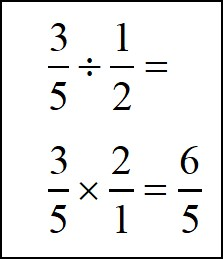##### Teaching Your Kids New Math, K-5 For Dummies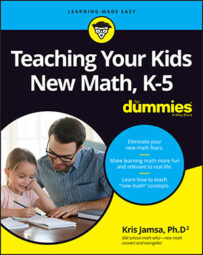When you’re working with your K–5 child to practice math skills, it can help to have a tool to remind you of some of the basics related to addition, subtraction, multiplication, division, and fractions. For those times, this Cheat Sheet can come in handy.

Practice daily with your addition and subtraction flash cards until your child can successfully complete timed addition or subtraction tests, which you can find online.

### Using a number line

For children just learning addition, both of small and large numbers, a visual aid, such as a number line, can be helpful. Your child should know that numbers on the number line increase from left to right. For younger grade levels, the number line will normally start at 0 or 1 and have an ending number at the far right.

Using a number line is similar to counting. For addition, you have a starting location and then you move right as you add a number. Again, for lower grades, you will typically move right one number at a time. For subtraction, you move left along the number line

For higher grades, however, the number line may not have starting and ending numbers. Instead, as your child performs addition or subtraction, they indicate a starting number to which they then add or subtract another number. In addition, depending on the numbers they are adding or subtracting, your child may move right or left by units of 100, 10, and finally 1.

The following figure illustrates using the number line for addition.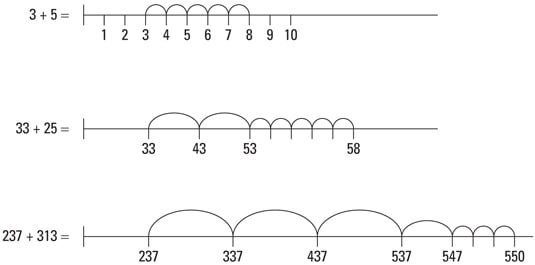The following illustrates the use of a number line for subtraction of small and large numbers.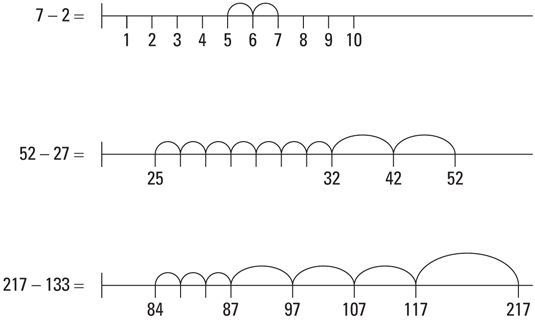Some time ago, students learned to add numbers using regrouping — a fancy term to describe carrying for addition and borrowing for subtraction. As your child begins to add numbers that require regrouping, you should draw a small box above the tens (or hundreds) digits into which your child will write the number they are carrying. The box will help your child better visualize the process of carrying a ten and will help them align the numbers they must add, as shown here: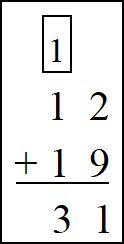A common new-math addition technique is to decompose large numbers into their hundreds, tens, and ones components and then separately add each. The following example illustrates how to use decomposition when adding large numbers: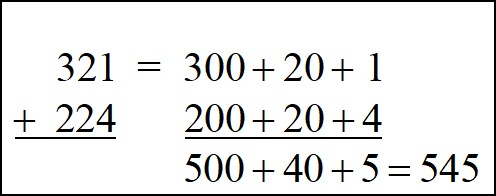### Subtracting with regrouping (borrowing)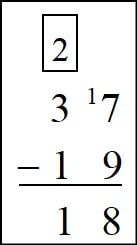### Checking results

When we use math, it’s like that we’ll eventually make a mistake. Fortunately, we can often detect mistakes if we take time to double-check our work.

One way to check work is by performing the complementary operation. For example, if your child is solving an addition problem, they can check their work using subtraction, as shown here: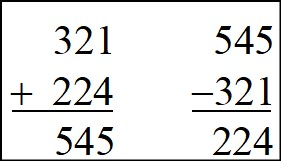If your child is working on a subtraction problem, they can check their work using addition, like so: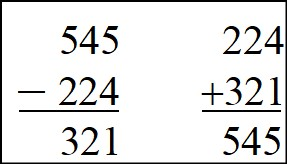## Multiplication and division

Practice daily with your multiplication and division flash cards until your child can successfully complete a timed multiplication or division test, which you can find online.

### Doing old school multiplication

To increase your child’s understanding and skills, have them practice multiplication problems using the old school approach, as shown here: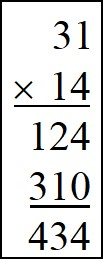### Doing Box Method multiplication

Another way to solve multiplication problems is with the Box Method, as shown in this example: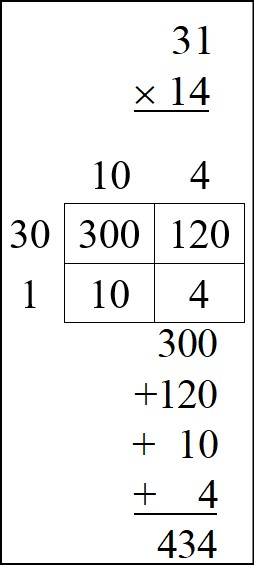### Long division example

Division problems with larger numbers require long division, as shown here: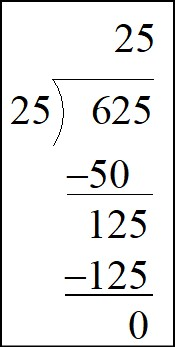### Checking results

Although this method is not often used, your child can check their work on a multiplication problem by using division, as in this example: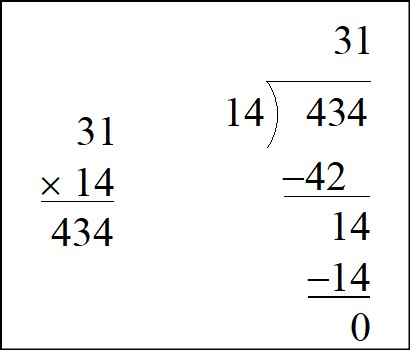They can check work on a division problem, by using multiplication, like so: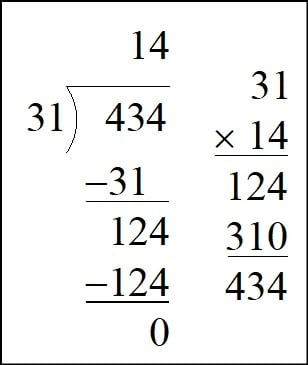## Fractions

Use the following examples as quick reminders for common fraction operations.

When adding like fractions (fractions in which the denominator, or bottom number, is the same), add the numerators (the top number). The denominator does not change, as shown here: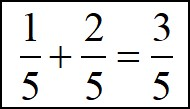When two fractions are unlike — the denominators are different — adding them is a slightly more involved process. Here are the steps for the following expression: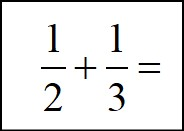1. Identify the common denominator by multiplying each fraction’s numerator and denominator by the denominator of the second fraction.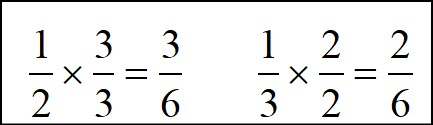1. Add the numerator (top number). The denominator does not change.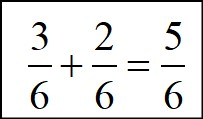### Subtracting like fractions

When subtracting like fractions (fractions in which the denominator, or bottom number, is the same), subtract the numerators (the top number). The denominator does not change, as shown here: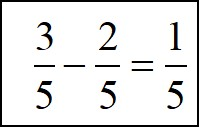### Subtracting unlike Fractions

When two fractions are unlike, you have to make the denominators the same before you can subtract the fractions: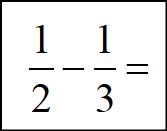1. Identify the common denominator by multiplying each fraction’s numerator and denominator by the denominator of the second fraction.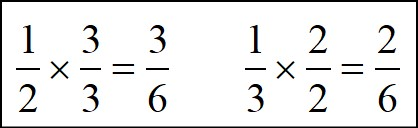1. Subtract the numerator (top number). The denominator does not change.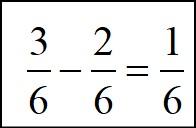### Multiplying fractions

When you multiply two fractions, you simply multiply the two numerators (top numbers) and also multiply the two denominators (bottom numbers), as shown here: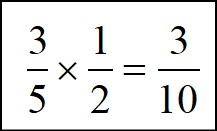### Dividing fractions

To divide fractions, flip the second fraction (to create its reciprocal) and then multiply: